# RD Sharma Solutions for Class 11 Chapter 33 - Probability Exercise 33.2

In this section, we will introduce an important term associated with a random experiment i.e., an event (“A subset of the sample space associated with a random experiment is called an event”). We shall also see how new events can be constructed by combining two or more events associated with a random experiment. The solutions are prepared by teachers having vast knowledge about the concepts covered in the exercise. Important formulas and shortcut tricks are provided by the subject experts to boost the exam preparation of students. Students can use RD Sharma Class 11 Maths Solutions as the best study material to revise the concepts before the exam and can also download the pdfs, from the links available below.

## Download the pdf of RD Sharma Solutions for Class 11 Maths Exercise 33.2 Chapter 33 – Probability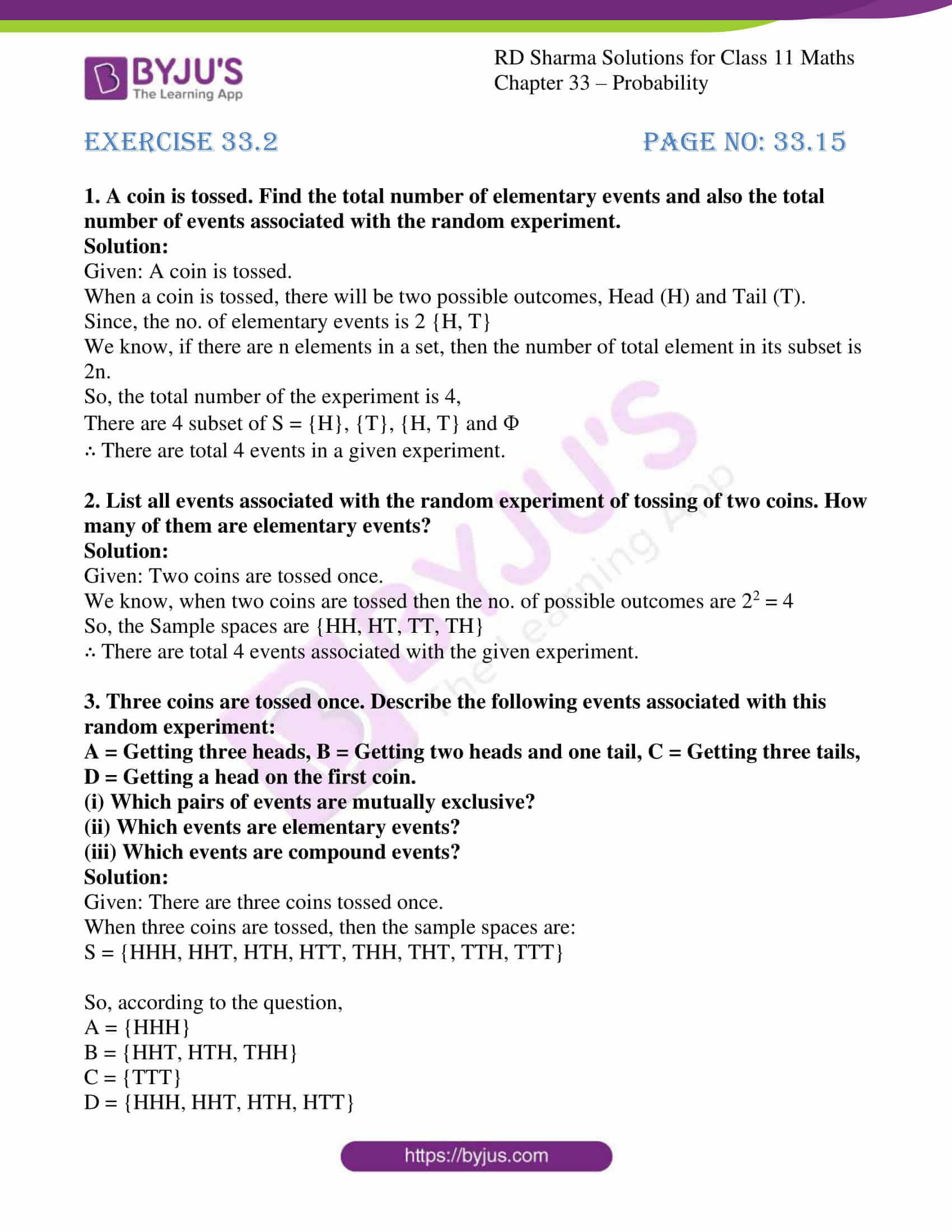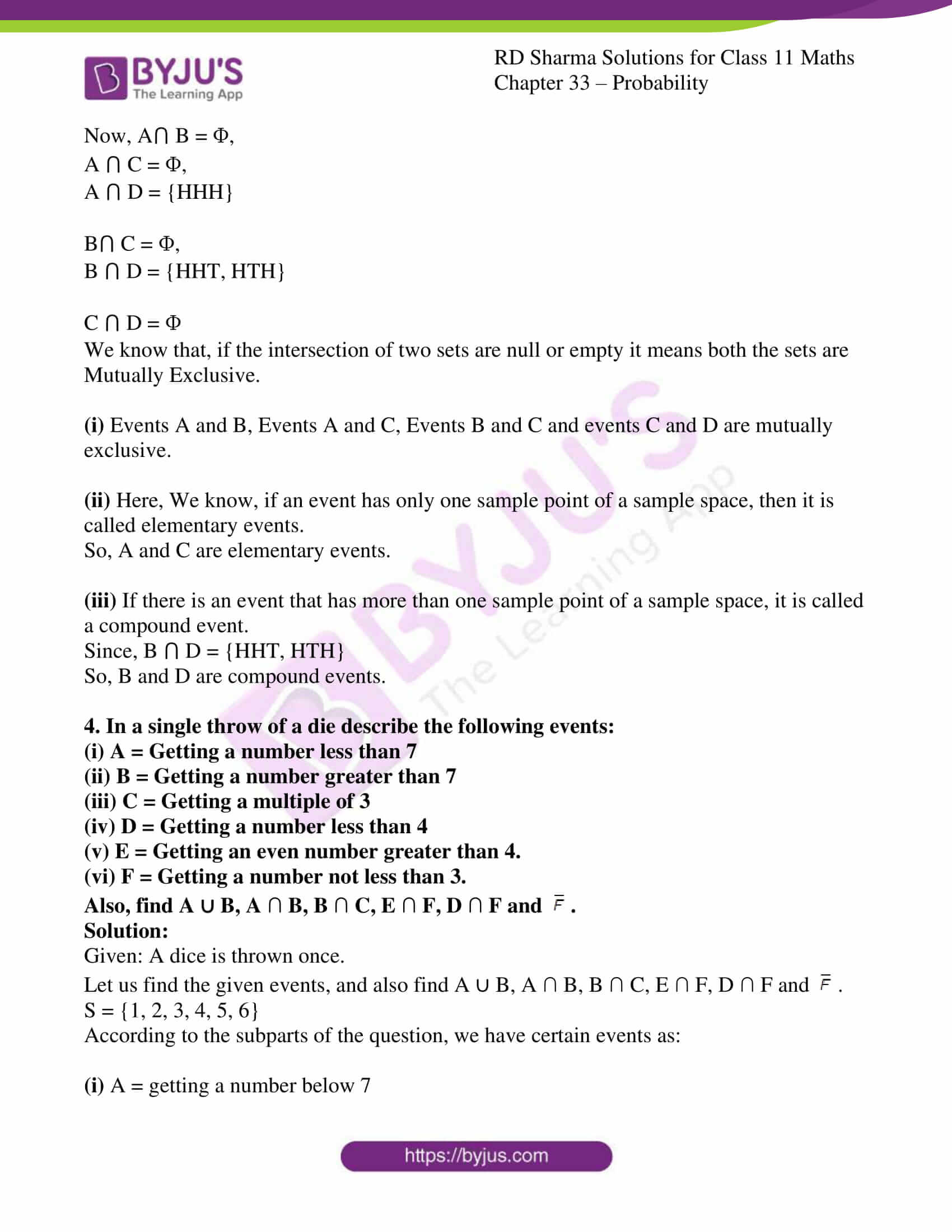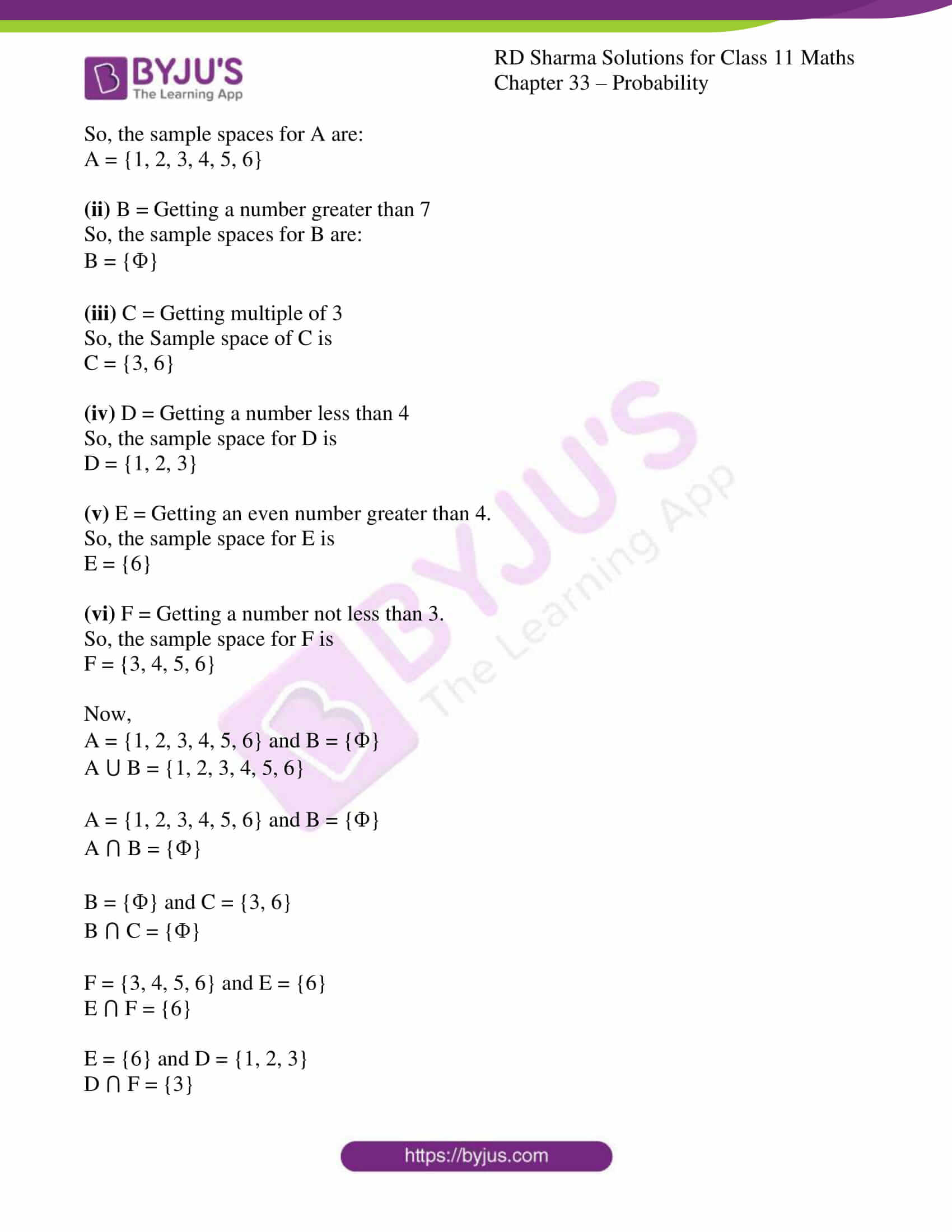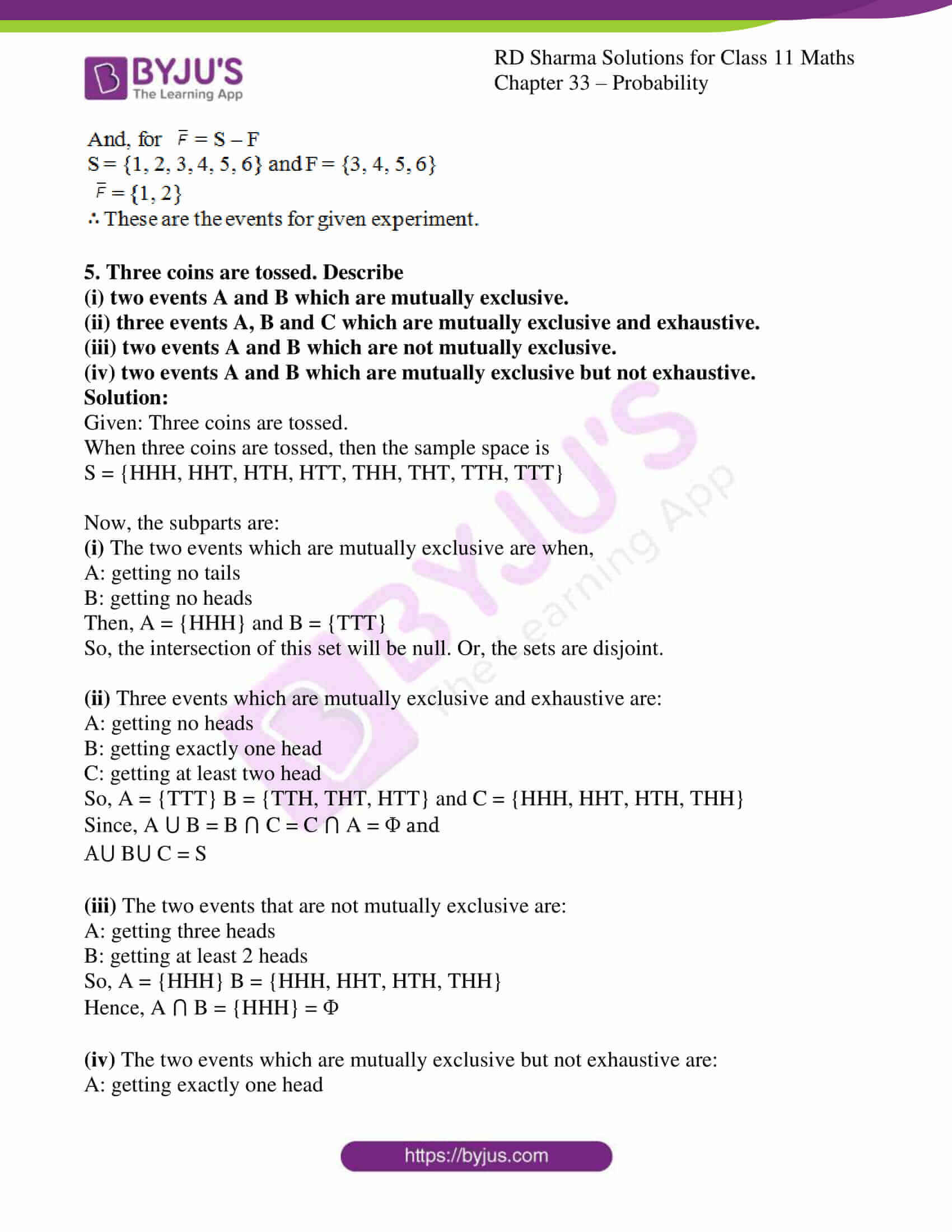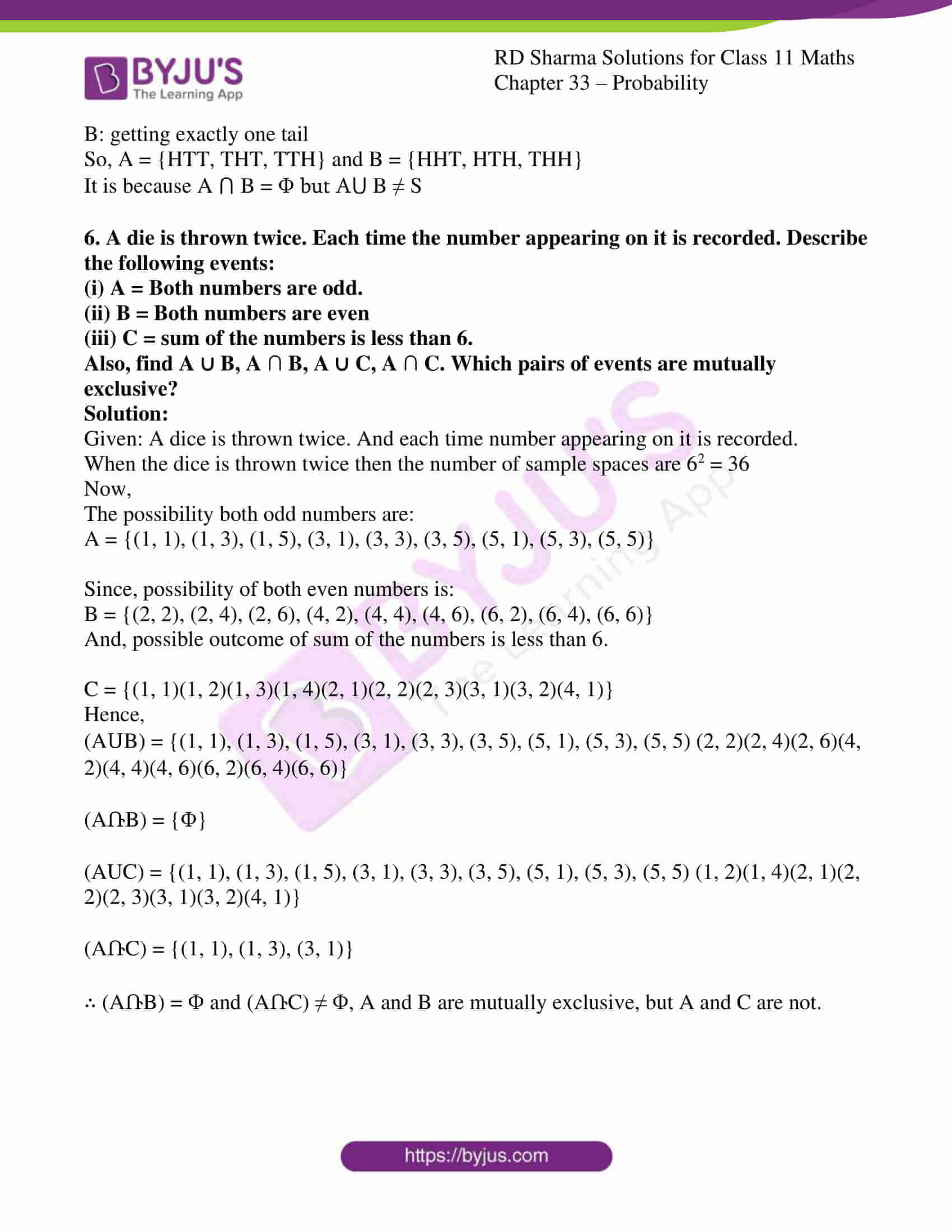### Also, access other exercises of RD Sharma Solutions for Class 11 Maths Chapter 33 – Probability

Exercise 33.1 Solutions

Exercise 33.3 Solutions

Exercise 33.4 Solutions

### Access answers to RD Sharma Solutions for Class 11 Maths Exercise 33.2 Chapter 33 – Probability

#### EXERCISE 33.2 PAGE NO: 33.15

1. A coin is tossed. Find the total number of elementary events and also the total number of events associated with the random experiment.

Solution:

Given: A coin is tossed.

When a coin is tossed, there will be two possible outcomes, Head (H) and Tail (T).

Since, the no. of elementary events is 2 {H, T}

We know, if there are n elements in a set, then the number of total element in its subset is 2n.

So, the total number of the experiment is 4,

There are 4 subset of S = {H}, {T}, {H, T} and Փ

∴ There are total 4 events in a given experiment.

2. List all events associated with the random experiment of tossing of two coins. How many of them are elementary events?

Solution:

Given: Two coins are tossed once.

We know, when two coins are tossed then the no. of possible outcomes are 22 = 4

So, the Sample spaces are {HH, HT, TT, TH}

∴ There are total 4 events associated with the given experiment.

3. Three coins are tossed once. Describe the following events associated with this random experiment:
A = Getting three heads, B = Getting two heads and one tail, C = Getting three tails, D = Getting a head on the first coin.
(i) Which pairs of events are mutually exclusive?
(ii) Which events are elementary events?
(iii) Which events are compound events?

Solution:

Given: There are three coins tossed once.

When three coins are tossed, then the sample spaces are:

S = {HHH, HHT, HTH, HTT, THH, THT, TTH, TTT}

So, according to the question,

A = {HHH}

B = {HHT, HTH, THH}

C = {TTT}

D = {HHH, HHT, HTH, HTT}

Now, A⋂ B = Փ,

A ⋂ C = Փ,

A ⋂ D = {HHH}

B⋂ C = Փ,

B ⋂ D = {HHT, HTH}

C ⋂ D = Փ

We know that, if the intersection of two sets are null or empty it means both the sets are Mutually Exclusive.

(i) Events A and B, Events A and C, Events B and C and events C and D are mutually exclusive.

(ii) Here, We know, if an event has only one sample point of a sample space, then it is called elementary events.

So, A and C are elementary events.

(iii) If there is an event that has more than one sample point of a sample space, it is called a compound event.

Since, B ⋂ D = {HHT, HTH}

So, B and D are compound events.

4. In a single throw of a die describe the following events:
(i) A = Getting a number less than 7
(ii) B = Getting a number greater than 7
(iii) C = Getting a multiple of 3
(iv) D = Getting a number less than 4
(v) E = Getting an even number greater than 4.
(vi) F = Getting a number not less than 3.
Also, find A ∪ B, A ∩ B, B ∩ C, E ∩ F, D ∩ F and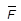.

Solution:

Given: A dice is thrown once.

Let us find the given events, and also find A ∪ B, A ∩ B, B ∩ C, E ∩ F, D ∩ F and.

S = {1, 2, 3, 4, 5, 6}

According to the subparts of the question, we have certain events as:

(i) A = getting a number below 7

So, the sample spaces for A are:

A = {1, 2, 3, 4, 5, 6}

(ii) B = Getting a number greater than 7

So, the sample spaces for B are:

B = {Փ}

(iii) C = Getting multiple of 3

So, the Sample space of C is

C = {3, 6}

(iv) D = Getting a number less than 4

So, the sample space for D is

D = {1, 2, 3}

(v) E = Getting an even number greater than 4.

So, the sample space for E is

E = {6}

(vi) F = Getting a number not less than 3.

So, the sample space for F is

F = {3, 4, 5, 6}

Now,

A = {1, 2, 3, 4, 5, 6} and B = {Փ}

A ⋃ B = {1, 2, 3, 4, 5, 6}

A = {1, 2, 3, 4, 5, 6} and B = {Փ}

A ⋂ B = {Փ}

B = {Փ} and C = {3, 6}

B ⋂ C = {Փ}

F = {3, 4, 5, 6} and E = {6}

E ⋂ F = {6}

E = {6} and D = {1, 2, 3}

D ⋂ F = {3}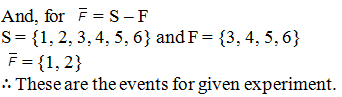5. Three coins are tossed. Describe
(i) two events A and B which are mutually exclusive.
(ii) three events A, B and C which are mutually exclusive and exhaustive.
(iii) two events A and B which are not mutually exclusive.
(iv) two events A and B which are mutually exclusive but not exhaustive.

Solution:

Given: Three coins are tossed.

When three coins are tossed, then the sample space is

S = {HHH, HHT, HTH, HTT, THH, THT, TTH, TTT}

Now, the subparts are:

(i) The two events which are mutually exclusive are when,

A: getting no tails

Then, A = {HHH} and B = {TTT}

So, the intersection of this set will be null. Or, the sets are disjoint.

(ii) Three events which are mutually exclusive and exhaustive are:

C: getting at least two head

So, A = {TTT} B = {TTH, THT, HTT} and C = {HHH, HHT, HTH, THH}

Since, A ⋃ B = B ⋂ C = C ⋂ A = Փ and

A⋃ B⋃ C = S

(iii) The two events that are not mutually exclusive are:

B: getting at least 2 heads

So, A = {HHH} B = {HHH, HHT, HTH, THH}

Hence, A ⋂ B = {HHH} = Փ

(iv) The two events which are mutually exclusive but not exhaustive are:

B: getting exactly one tail

So, A = {HTT, THT, TTH} and B = {HHT, HTH, THH}

It is because A ⋂ B = Փ but A⋃ B ≠ S

6. A die is thrown twice. Each time the number appearing on it is recorded. Describe the following events:
(i) A = Both numbers are odd.
(ii) B = Both numbers are even
(iii) C = sum of the numbers is less than 6.
Also, find A ∪ B, A ∩ B, A ∪ C, A ∩ C. Which pairs of events are mutually exclusive?

Solution:

Given: A dice is thrown twice. And each time number appearing on it is recorded.

When the dice is thrown twice then the number of sample spaces are 62 = 36

Now,

The possibility both odd numbers are:

A = {(1, 1), (1, 3), (1, 5), (3, 1), (3, 3), (3, 5), (5, 1), (5, 3), (5, 5)}

Since, possibility of both even numbers is:

B = {(2, 2), (2, 4), (2, 6), (4, 2), (4, 4), (4, 6), (6, 2), (6, 4), (6, 6)}

And, possible outcome of sum of the numbers is less than 6.

C = {(1, 1)(1, 2)(1, 3)(1, 4)(2, 1)(2, 2)(2, 3)(3, 1)(3, 2)(4, 1)}

Hence,

(AՍB) = {(1, 1), (1, 3), (1, 5), (3, 1), (3, 3), (3, 5), (5, 1), (5, 3), (5, 5) (2, 2)(2, 4)(2, 6)(4, 2)(4, 4)(4, 6)(6, 2)(6, 4)(6, 6)}

(AՌB) = {Փ}

(AUC) = {(1, 1), (1, 3), (1, 5), (3, 1), (3, 3), (3, 5), (5, 1), (5, 3), (5, 5) (1, 2)(1, 4)(2, 1)(2, 2)(2, 3)(3, 1)(3, 2)(4, 1)}

(AՌC) = {(1, 1), (1, 3), (3, 1)}

∴ (AՌB) = Փ and (AՌC) ≠ Փ, A and B are mutually exclusive, but A and C are not.